## ML Aggarwal Class 10 Solutions for ICSE Maths Chapter 9 Arithmetic and Geometric Progressions Ex 9.1

These Solutions are part of ML Aggarwal Class 10 Solutions for ICSE Maths. Here we have given ML Aggarwal Class 10 Solutions for ICSE Maths Chapter 9 Arithmetic and Geometric Progressions Ex 9.1

More Exercises

Question 1.
For the following A.P.s, write the first term a and the common difference d:
(i) 3, 1, – 1, – 3, …
(ii) $$\frac { 1 }{ 3 } ,\frac { 5 }{ 3 } ,\frac { 9 }{ 3 } ,\frac { 13 }{ 3 } ,….$$
(iii) – 3.2, – 3, – 2.8, – 2.6, …
Solution:
(i) 3, 1, -1, -3, …
Here first term (a) = 3
and the common difference (d)
= 1 – 3 = -2,
– 1 – 1 = -2,…
= -2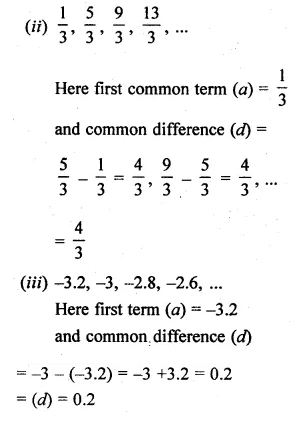Question 2.
Write first four terms of the A.P., when the first term a and the common difference d are given as follows :
(i) a = 10, d = 10
(ii) a = – 2, d = 0
(iii) a = 4, d = – 3
(iv) a = $$\\ \frac { 1 }{ 2 }$$, d = $$– \frac { 1 }{ 2 }$$
Solution:
(i) a = 10, d = 10
∴ A.P. = 10, 20, 30, 40, …
(ii) a = -2, d = 0
∴ A.P. = -2, -2, -2, -2, ….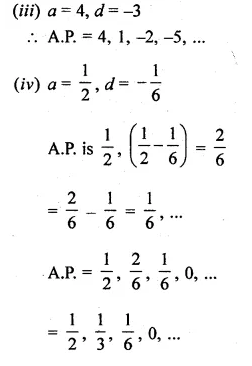Question 3.
Which of the following lists of numbers form an A.P.? If they form an A.P., find the common difference d and write the next three terms :
(i) 4, 10, 16, 22,…
(ii) – 2, 2, – 2, 2,…..
(iii) 2, 4, 8, 16,….
(iv) 2, $$\\ \frac { 5 }{ 2 }$$, 3, $$\\ \frac { 7 }{ 2 }$$,……
(v) – 10, – 6, – 2, 2,….
(vi) 1², 3², 5², 7²,….
(vii) 1, 3, 9, 27,….
(viii) √2, √8, √18, √32,….
(ix) 3, 3 + √2, 3 + √2, 3 + 3√2,…..
(x) √3, √6, √9, √12,……
(xi) a, 2a, 3a, 4a,…….
(xii) a, 2a + 1, 3a + 2, 4a + 3,….
Solution:
(i) 4, 10, 16, 22,…
Here a = 4, d = 10 – 4 = 6, 16 – 10 = 6, 22 – 16 = 6
∵ common difference is same
∵ It is in A.P
and next three terms are 28, 34, 40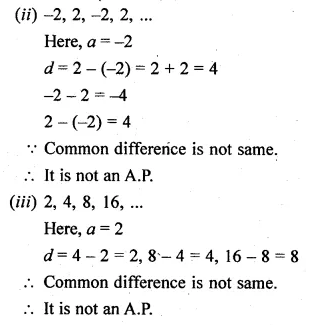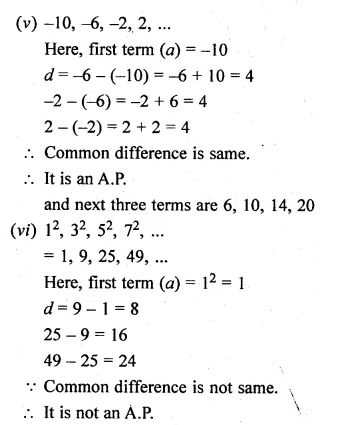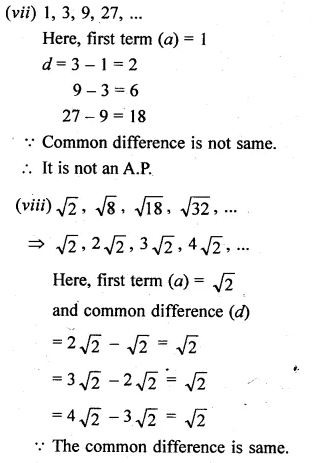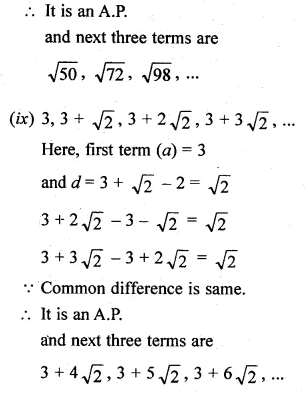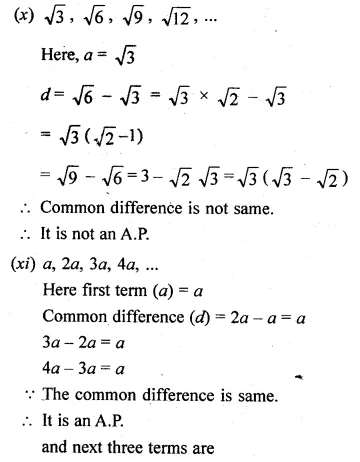Hope given ML Aggarwal Class 10 Solutions for ICSE Maths Chapter 9 Arithmetic and Geometric Progressions Ex 9.1are helpful to complete your math homework.

If you have any doubts, please comment below. Learn Insta try to provide online math tutoring for you.